# Short Run Xbar-s Charts

Short run Xbar and s (Xbar-s) charts can help you identify changes in the averages and standard deviation of multiple characteristics in a limited production run. Review the following example—an excerpt from Innovative Control Charting1—to get a sense of how a short run Xbar-chart works.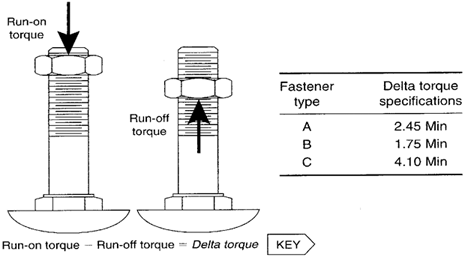Figure 1. Delta torque is a performance key characteristic on self-locking fastener systems.

## Case Description

Torque is tested on self-locking nuts using precision stud standards and production nuts. During production, the nuts are slightly deformed so that the threads create an interference or locking fit with the stud. The run-on torque is the average prevailing torque while turning the nut on the stud seven clockwise revolutions. The runoff torque is the maximum force it takes to turn the nut back off the stud one counterclockwise revolution. The delta torque is the run-on torque minus the run-off torque. Each fastening system has its own minimum delta torque requirements and the standard deviations are expected to vary from system to system.

## Sampling Strategy

Torque tests are performed for each batch of locking nuts. Ten samples are tested from each batch. To monitor the delta torque consistency, regardless of the nut/bolt locking system, a short run Xbar-s chart is selected. This is the appropriate chart because the subgroup sizes are large and the standard deviations are different from system to system.

## Target Values

Before a short run chart can be used, target values must first be defined.

### Locking System A

System A has previously been maintained using traditional Xbar-s charts. On the most recent set of in-control charts, the centerline on the Xbar chart was 2.920. The centerline on the s chart was 0.089. Therefore, these centerlines are used as target values for system A.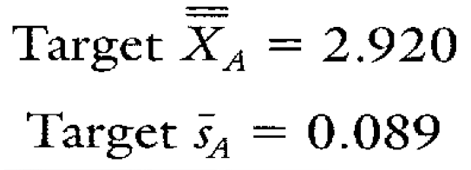Figure 2. Target values for locking system A.

### Locking System B

The consistency of locking system B has never been evaluated with a control chart. However, quality assurance personnel have taken 28 delta torque measurements at some time in the past. Equation 15.14 was used to convert the sample standard deviation from those 28 measurements into the targets found in Figure 3.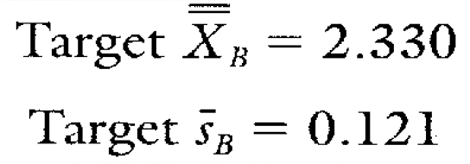Figure 3. Target values for locking system B.

### Locking System C

Like system A, Rocking system C has previously been evaluated using traditional Xbar-s charts. On the most recent set of in-control charts, the centerline on the Xbar chart was 5.125. The centerline on the s chart was 0.337. Therefore, these centerlines are used as target values for system C (see Figure 4).Figure 4. Target values for locking system C.

## Data Collection Sheet

Table 1. Delta torque data sheet and plot point calculations.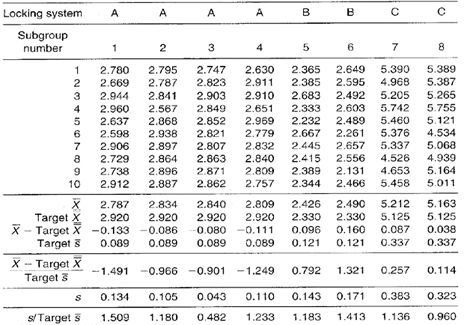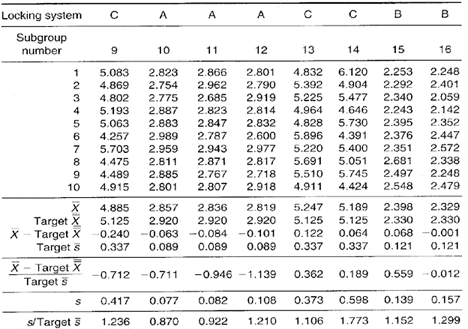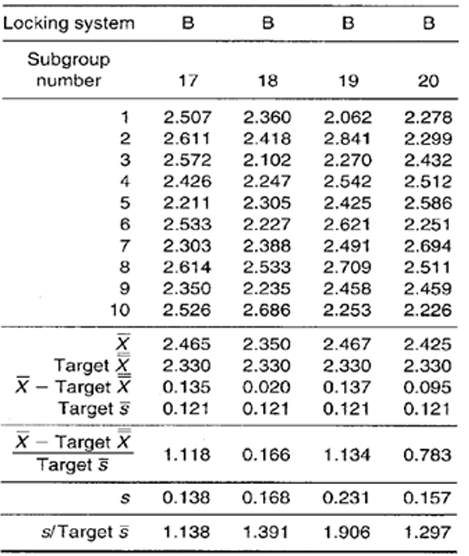## Short Run Xbar-s Chart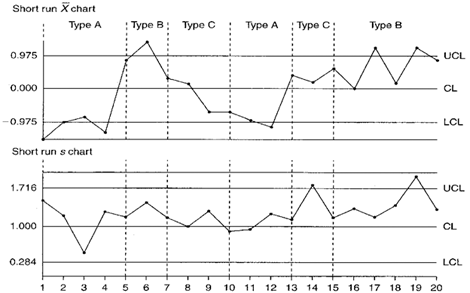Figure 5. Delta torque short run Xbar and s control charts for locking systems A, B, and C.

## Chart Interpretation

Short run s chart: If evaluating product-specific variation, locking system A’s delta torque seems to be behaving randomly. All eight of system B’s plot points fall above the centerline with one of them falling above the UCL. System C’s delta torque favors the high side with one plot point beyond the UCL. Overall, the process reveals a run of 9 plot points above the centerline that occur across three product lines (subgroups 13 through 20).

Short run Xbar chart: All seven of system A’s plot points fall below the centerline with three of them falling below the LCL. Seven of system B’s eight plot points are situated above the centerline with three above the UCL. System C appears to be behaving randomly. Looking at patterns across locking systems, there is a gradual decrease in the average from plot point 6 through 12. Also, it looks as though the average has shifted higher between plot points 13 and 20.

## Recommendations

Note: Plot point patterns above and below the centerlines and beyond the control limits are present, but the action to take depends entirely on how the target values were estimated.

### Locking System A

Short run s chart: The target s came from past control charts, therefore, the fact that the plot points are behaving randomly indicates that the standard deviation has not changed since data were last recorded.

Short run Xbar chart: The target X came from past charts, therefore, the run below the centerline indicates the delta torque has decreased since data were last recorded. This is an assignable cause and should be investigated. If the shift is found to be desirable, deliberate, and permanent, the target X should be recalculated based on system A’s current overall average. If the shift is found to be an unwanted condition, do not recalculate target X. Instead, eliminate the cause of the downward shift.

### Locking System B

Short run s chart: The target s came from past quality assurance records. The run above the centerline, therefore, indicates that the standard deviation has significantly increased since data were last recorded. This may be an assignable cause and should be investigated. If the shift is found to be an unwanted condition, do not recalculate target s. Instead, eliminate the cause of the increased variability.

Short run Xbar chart: The target X came from quality assurance records, therefore, the run above the centerline indicates the delta torque has increased since data were last recorded. This may be an assignable cause and should be investigated. If this significant increase in delta torque is desirable, then the target X should be recalculated based on system B’s current overall average. If the shift is unwanted, do not recalculate target X. Instead, eliminate the assignable cause for the increase in the delta torque average.

### Locking System C

Short run s chart: Because the target s was based on the centerline from an older, in-control s chart, the run above the centerline indicates that the process standard deviation has increased significantly since the last time the system C product was manufactured. This should be treated as an assignable cause because the target s is based upon actual data. If the increase in standard deviation for system C is expected to be a permanent change, then the target s should be recalculated based on the current overall average standard deviation (see Calculation 1). Otherwise, if the assignable cause is to be removed to reduce the current amount of variation, the old target s should be saved to represent the current expected level of variability.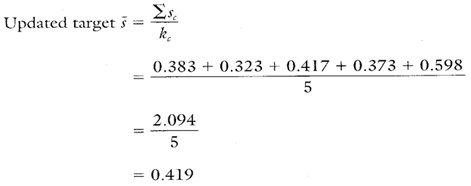Calculation 1. Recalculating locking system C's target s based on current data from control chart. This is done only if the change in variability is expected to be a permanent one.

Short run Xbar chart: The target X has been obtained from a recent in-control chart, and the plot points are behaving randomly. This indicates that the initial target X was a good estimator of the actual delta torque. There is no need to recalculate system C’s target X.

## Estimating the Process Average

Estimates of the process average should be calculated separately for each characteristic or part on short run Xbar-s charts. In this case, estimates of the process average should be calculated separately for each different locking system. Calculation 2 shows the calculation for the estimate of the overall average of locking system B.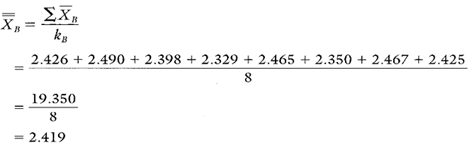Calculation 2. Estimate of the process average for locking system B.

## Estimating Sigma

Estimates of sigma are also calculated separately for each characteristic or location represented on short run Xbar-s charts. In this case, estimates of the process standard deviation should be calculated for each different locking system. Estimates of the process standard deviation for locking system B are found in Calculation 3.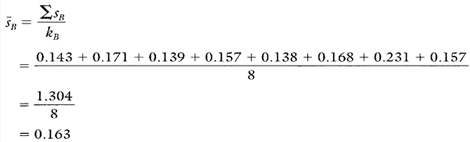Calculation 3. Calculation of s for locking system B based on current data from the short run s control chart.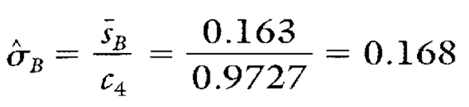Calculation 4. Calculation of the estimate of the process standard deviation for locking system B.
Note: To ensure reliable estimates, k needs to be at least 20. In this example, k is only 8. Therefore, the estimates here and in Table 2 are used for illustration purposes only.

## Calculating Process Capability and Performance Ratios

The Cpk lower calculation for locking system B is shown in Calculation 5. Because there is only a minimum specification, no Cp or Cpk upper value is calculated for locking system B.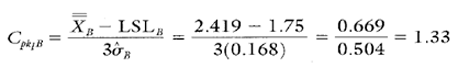Calculation 5. Cpk lower calculation for fastener system B delta torque.

## Short Run Xbar-s Chart Advantages

• Graphically illustrates the variation of multiple products with different nominals, different standard deviations, and even different units of measure all on the same chart.
• Separates sources of process variability from sources of product variability.
• Due to the large sample sizes, the short run Xbar chart is sensitive to small changes in the process average.
• Summaries large amounts of data.

## Short Run Xbar-s Chart Disadvantages

• Requires software to effectively handle large amounts of data.
• The use of negative numbers and unitless ratios may be confusing at first.
• X, s, and process standard deviation estimates must be calculated separately for each characteristic represented on the chart.

• The process capability and performance ratio calculations for locking systems A and C are found in Table 2.
• Summary statistics and Cpk lower values for systems A and C are based on the actual data from the data collection sheet (Table 1). In addition, no Cp or Cpk upper values are found in Table 2 because the locking systems all have one-sided specifications.

Table 2. Additional summary statistics and process performance ratios for locking systems A and C.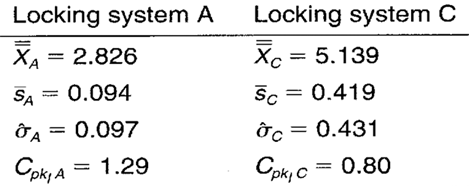When you use SPC software from InfinityQS, consuming the information provided by short run Xbar-charts becomes faster and easier than ever. See how this type of analysis is surfaced in InfinityQS solutions.

FOOTNOTE:
1 Wise, Stephen A. and Douglas C. Fair. Innovative Control Charting: Practical SPC Solutions for Today’s Manufacturing Environment. Milwaukee, WI: ASQ Quality Press.

### Take the first step from quality to excellence

Take the Next Steps